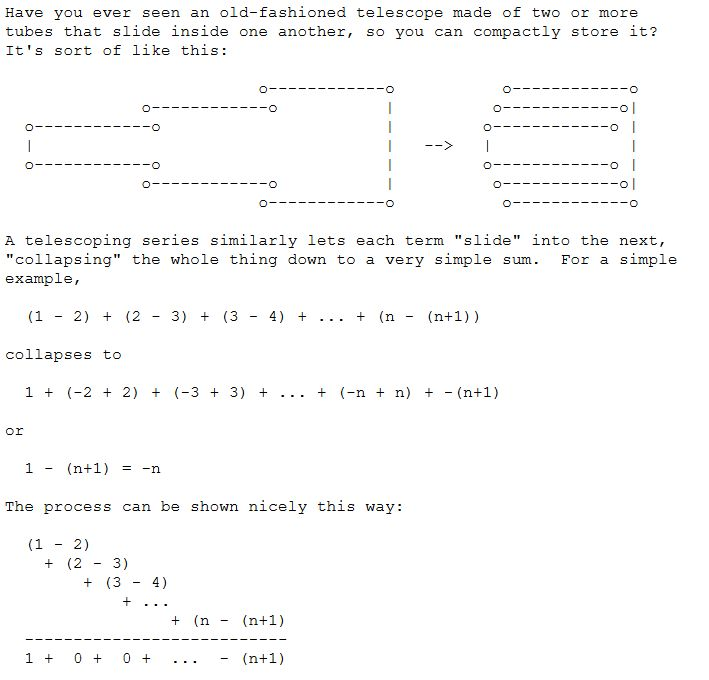Show Question
Math and science::Analysis::Tao::07. Series

# Telescoping series

Let $$(a_n)_{n=0}^{\infty}$$ be a sequence of real numbers which converge to 0, i.e., $$lim_{n \rightarrow \infty} a_n = 0$$. Then the series $$\sum_{n=0}^{\infty}(a_n - a_{n+1})$$ converges to $$a_0$$.

A post on The Math Forum talks about the naming of this series:p170